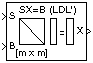# LDL Solver

Solve SX=B for X when S is square Hermitian positive definite matrix

## Library

Math Functions / Matrices and Linear Algebra / Linear System Solvers

`dspsolvers`

•## Description

The LDL Solver block solves the linear system SX=B by applying LDL factorization to the matrix at the S port, which must be square (M-by-M) and Hermitian positive definite. Only the diagonal and lower triangle of the matrix are used, and any imaginary component of the diagonal entries is disregarded. The input to the B port is the right side M-by-N matrix, B. The M-by-N output matrix X is the unique solution of the equations.

A length-M unoriented vector input for right side B is treated as an M-by-1 matrix.

When the input is not positive definite, the block reacts with the behavior specified by the Non-positive definite input parameter. The following options are available:

• `Ignore` — Proceed with the computation and do not issue an alert. The output is not a valid solution.

• `Warning` — Proceed with the computation and display a warning message in the MATLAB® Command Window. The output is not a valid solution.

• `Error` — Display an error dialog and terminate the simulation.

Note

The Non-positive definite input parameter is a diagnostic parameter. Like all diagnostic parameters on the Configuration Parameters dialog, it is set to `Ignore` in the code generated for this block by Simulink® Coder™ code generation software.

## Algorithm

The LDL algorithm uniquely factors the Hermitian positive definite input matrix S as

S = LDL*

where L is a lower triangular square matrix with unity diagonal elements, D is a diagonal matrix, and L* is the Hermitian (complex conjugate) transpose of L.

The equation

LDL*X = B

is solved for X by the following steps:

1. Substitute

Y = DL*X

2. Substitute

Z = L*X

3. Solve one diagonal and two triangular systems.

LY = B

DZ = Y

L*X = Z

## Parameters

Non-positive definite input

Response to nonpositive definite matrix inputs.

## Supported Data Types

• Double-precision floating point

• Single-precision floating point